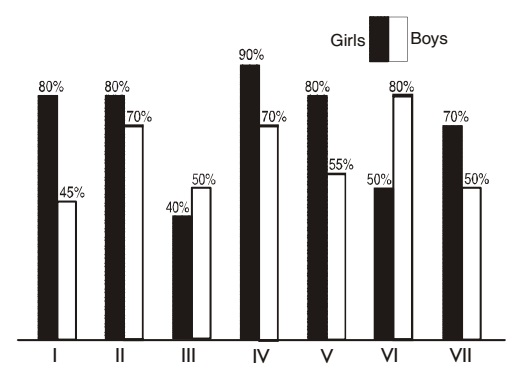## Bar graph

#### Data Interpretation

Direction: The bar graph given below shows annual result of different classes of a school. Study the graph carefully and attempt the question that follow:1. In which class pass percentage of boys is more than the average pass percentage of girls of the school?

1. Average pass percentage of girls = Total number of pass girls in school/7

##### Correct Option: A

Average pass percentage of girls = Total number of girls for pass the class/7
Average pass percentage of girls = 80 + 80 + 40 + 90 + 80 + 50 + 70/7 = 490/7 = 70%
Therefore. in class VI pass percentage of boys is more than the average pass percentage of girls.

1. In which class number of failed girls is minimum?

1. In class IV pass percentage of girls is the highest.

##### Correct Option: C

In class IV pass percentage of girls is the highest. Thereore in this class only number of failed girls is the minimum.

1. Result of which class shows maximum difference between pass percentage of the girls and the boy?

1. Calculate the difference between pass percentage of girls and boys in classes.

##### Correct Option: A

Difference between pass percentage of girls and boys in class I = 80 - 45 = 35%
Difference between pass percentage of girls and boys in class II = 80 - 70 = 10%
Difference between pass percentage of girls and boys in class III = 50 - 40 = 10%
Difference between pass percentage of girls and boys in class IV = 90 - 70 = 20%
Difference between pass percentage of girls and boys in class V = 80 - 55 = 25%
Difference between pass percentage of girls and boys in class VI = 80 - 50 = 30%
Difference between pass percentage of girls and boys in class VII = 70 - 50 = 20%
Hence, it is clear that result of class I shows maximum difference between pass percentage of the girls and the boys.

1. Average pass percentage of boy in classes II and III is equal to average pass percentage of girls in which of the following classes?

1. As per graph
Average pass percentage of the boys in classes II and III = 70 + 50/2 = 120/2 = 60%
Try option one by one and solve the question.

##### Correct Option: C

As per graph
Average pass percentage of the boys in classes II and III = 70 + 50/2 = 120/2 = 60%
As per given graph we can find that
Average pass percentage of the boys in classes VI and Vii = 50 + 70/2 = 60%
Therefore. average pass percentage of boys in classes II and III is equal to the average pass percentage of girls in classes VI and VII.

1. In which of the following classes pass percentage of girls is less than the average pass percentage of the school?

1. Average pass percentage of the school = Total number of pass Boys and girls / 14

##### Correct Option: A

Average pass percentage of the school = Total number of pass Boys and girls / 14
Average pass percentage of the school = (80 + 45 + 80 + 70 + 40 + 50 + 90 + 70 + 80 + 55 + 50 + 80 + 70 + 50/14 = 910)/14 = 65%
Therefore, it is clear that pass percentage of the girls in III and VI classes is less than the average pass percentage of the school.